Courses
Courses for Kids
Free study material
Free LIVE classes
More

# Application of Pythagorean Theorem in Daily LifeLIVE
Join Vedantu’s FREE Mastercalss

## An Introduction to Pythagorean Theorem

The Pythagorean theorem also known as the Pythagoras theorem explains “the relationship between the three sides of a right-angled triangle which are also referred to as the Pythagorean Triplet”.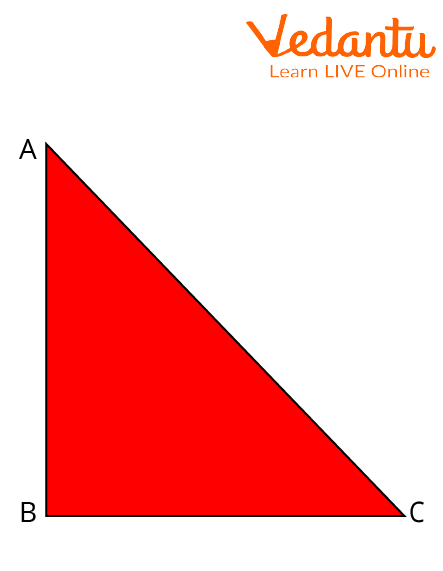Right-Angled Triangle

In the figure shown above, $ABC$ is a right-angle triangle with right angled at $B$ and $AC$ in the hypotenuse, $BC$ is the base for angle $C$ and $AB$ is perpendicular. $\underline{\underline{\text { So }}}$ in this Triangle according to Pythagoras' Theorem:

$(A C)^{2}=(A B)^{2}+(BC)^{2}$

In this article, we will be discussing the real-life application of the Pythagoras theorem, i.e., the application of the theorem in our daily life.

## History of Mathematician

The Pythagorean theorem takes its name from the ancient Greek mathematician Pythagoras.Scientist Pythagoras

• Name: Pythagoras

• Field: Mathematics and Philosopher

• Nationality: Greek

## Statement of Pythagoras Theorem

Pythagoras theorem states that In a right-angled triangle, the square of the hypotenuse side is equal to the sum of squares of the perpendicular and the base, i.e., the other sides of the triangle.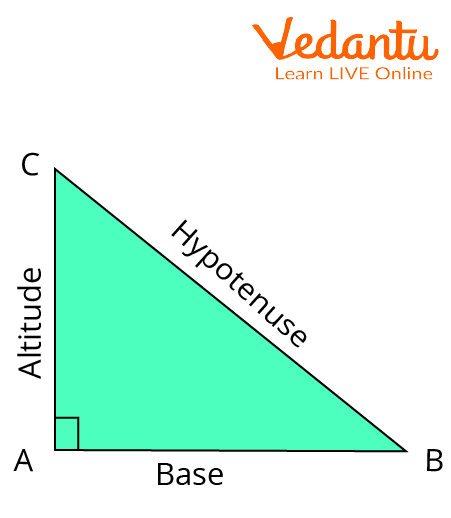Statement of Pythagoras Theorem

In the above triangle, for angle $B, B C$ is the hypotenuse, $A B$ is the base, and $A C$ is the perpendicular. So,

$(B C)^{2}=(A B)^{2}+(AC)^{2}$

Note: Hypotenuse is the longest side of the right-angle triangle.

## The Formula of Pythagoras Theorem

$(\text { Hypotenuse })^{2}=(\text { Base })^{2}+(\text { Perpendicular })^{2} \\$

$(B C)^{2}=(A B)^{2}+(AC)^{2}$

where BC is hypotenuse, AB is base, and AC is perpendicular for right-angled triangle ABC.

## Proof of Pythagoras Theorem

• Given: A right-angled triangle $\mathrm{ABC}$, right-angled at $\mathrm{B}$.

• To Prove: $A C^{2}=A B^{2}+B C^{2}$

• Construction: Draw a perpendicular BD meeting $A C$ at $D$.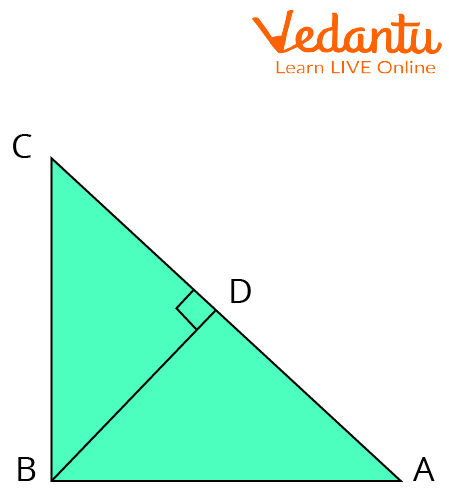Construction to Prove Pythagoras Theorem

We know $\triangle A D B \sim \triangle A B C$

Therefore,

$\dfrac{A D}{A B}=\dfrac{A B}{A C}$

(Corresponding sides of similar triangles)

Or, $A B^{2}=A D \times A C$

Also, $\triangle B D C \sim \triangle A B C$

Therefore,

$\dfrac{C D}{B C}=\dfrac{B C}{A C}$

(Corresponding sides of similar triangles)

or, $B C^{2}=C D \times A C$

Adding the equations (1) and (2), we get,

$A B^{2}+B C^{2}=A D \times A C+C D \times A C$

$\Rightarrow A B^{2}+B C^{2}=A C(A D+C D)$

Since $A D+C D=A C$

Therefore, $A C^{2}=A B^{2}+B C^{2}$

Hence, we proved Pythagoras theorem.

Now, Let us discuss the converse of Pythagoras Theorem with detailed proof.

## Converse of Pythagoras Theorem and Its Proof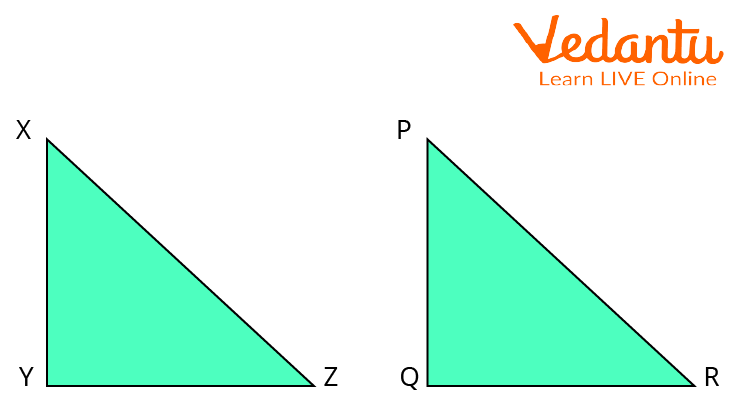Two Right-Angled Similar Triangles

• In a triangle, if the square of one side is equal to the sum of the other two sides, then the angle opposite the first side is a right angle.

• Given: $\ln \Delta X Y Z, X Y^{2}+Y Z^{2}=X Z^{2}$

• To prove: $\angle X Y Z=90^{\circ}$

• Construction: A triangle PQR is constructed such that a triangle $P Q R$ is constructed such that

$P Q=X Y, Q R=Y Z \angle P Q R=90^{\circ}$

Proof: $\ln \triangle P Q R, \angle Q=90^{\circ}$

$P R^{2}=P Q^{2}+Q R^{2}$ {Pythagoras theorem}

or $P R^{2}=X Y^{2}+Y Z^{2} \ldots$ (i) $[P Q=X Y, Q R=Y Z]$

But we know $X Z^{2}=X Y^{2}+Y Z^{2} \ldots \ldots$ (ii) (given)

Therefore, $X Z^{2}=P R^{2}$ {From equation (i) and (ii)}

or $X Z=P R$

or $\triangle X Y Z \cong \triangle P Q R$ {SSS congruence rule}

Therefore, $\angle Y=\angle Q=90^{\circ}$ {CPCT}

Hence, $\angle X Y Z=90^{\circ}$

The converse of Pythagoras theorem is proved.

## Application of Pythagoras Theorem

• Pythagoras Theorem is used to find the steepness of hills.

• In Artificial intelligence: face recognition features in security cameras use the Pythagorean theorem. The distance between the camera and the person is recorded.

• Pythagoras Theorem is used to find the shortest distance in Navigation.

• The concept of the Pythagoras Theorem is also used in Interior Designing.

• Pythagoras theorem is used to find the third side of a right-angled triangle when 2 sides are given.

• In Engineering, the diameter can be easily calculated when length and breadth are known using Pythagoras Theorem.

## Limitations

• Pythagoras Theorem is only applicable in the case of the Right-Angled Triangle. If one of the angles is not 90 degrees, then we cannot use Pythagoras Theorem.

• Pythagoras Theorem is only useful in the case of 2-dimensional figures. It cannot be applied in the case of 3-dimensional figures or objects.

## Solved Examples

1. The perpendicular of a right-angled triangle is given as 12cm and the hypotenuse is given as 13cm. Find the base of the given triangle.

Solution:

Perpendicular $=12 \mathrm{~cm}$

Base $=b \mathrm{~cm}$

Hypotenuse $=13 \mathrm{~cm}$

As per the Pythagorean Theorem, we have

$Perpendicular^{2}+ Base^{2}= Hypotenuse^{2}$

$\Rightarrow 12^{2}+b^{2}=13^{2}$

$\Rightarrow 144+b^{2}=169$

$\Rightarrow b^{2}=169-144$

$\Rightarrow b^{2}=25$

$\Rightarrow b=\sqrt{25}$

Therefore, $b=5 \mathrm{~cm}$

2. The sides of a triangle are 15, 17, and 8 units. Check if it has a right angle or not.

Solution: Clearly, 17 is the longest side.

It also satisfies the condition $15+8>17$

We know

$c^{2}=a^{2}+b^{2}$

So, let $a=15, b=8$, and $c=17$

First, we will solve R.H.S. of Equation (1).

$a^{2}+b^{2}=15^{2}+8^{2}=225+64=289$

Now, taking L.H.S, we get

$c^{2}=17^{2}=289$

We can see LHS=RHS.

Therefore, the given triangle is a right triangle, as it satisfies the Pythagoras theorem.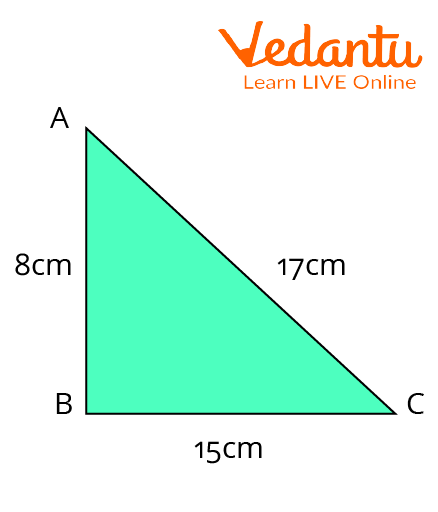To Check Angle of Triangle

## Important Formulas

For any Right-Angled Triangle,

$(\text { Hypotenuse })^{2}=(\text { Base })^{2}+(\text { Perpendicular })^{2}$

## Important Points to Remember

• Pythagoras theorem is applicable only in case of a right-angled triangle.

• Trigonometry is based on the Pythagoras Theorem.

## Conclusion

In this article, we discussed the Pythagoras Theorem in depth. The proof, statement, applications, and examples are thoroughly explained above. From the discussion above about the Pythagoras theorem, we can conclude that it is a very useful theorem to be used in solving questions of a right-angle triangle and it helps us in real life a lot.

Last updated date: 18th Sep 2023
Total views: 102.3k
Views today: 2.02k

## FAQs on Application of Pythagorean Theorem in Daily Life

1. Discuss some important facts about Pythagoras's triples?

Some important facts are listed below:

• Pythagoras' Triplets cannot have decimals. They can only be Natural Numbers.

• There is only one triple with three consecutive number pairs, i.e., $(3,4,5)$.

• All triples in Arithmetic Progression are multiples of $(3,4,5)$.

• The multiples of Pythagoras triple $(5,12,13)$ again gives us a unique Pythagoras Triple.

• A Pythagorean Triple can never be made of all odd numbers or two even numbers and one odd number.

• There are 16 groups of Pythagorean triples under $100 .$

2. Can the Pythagorean Theorem Formula be applied to any triangle?

No, Pythagoras Theorem can only be applied to right-angled triangles. The relation between the sides of a triangle, i.e., the sum of the square of two sides is equal to the square of the third side is satisfied only in a right-angled triangle. For acute and obtuse angled triangles, the sum of squares of two sides of a triangle is smaller and greater than the square of the third side, respectively. So, Pythagoras Theorem can not be applied in that case.

3. What are the different ways to prove Pythagoras's theorem?

There are different ways to prove Pythagoras' Theorem. There are 371 proofs of Pythagoras Theorem. Some of them are discussed below:

• We can prove it using the properties of similar triangles.

• Euclid’s proof can also be used to prove the Pythagorean Theorem.

• We can also prove it using Differential Equations.

• With the help of Algebraic Proofs Pythagorean Theorem can also be proved.

• Einstein's proof by dissection without rearrangement can also be used to prove the theorem and so on.

4. How did the Pythagorean theorem change the world?

The Pythagoras theorem has changed the world with its massive applications in Mathematics, Science, and technology. For the past 2500-2500 years, the Pythagoras theorem, arguably the utmost well-known theorem worldwide, has significantly been useful to mankind. Its beneficial right angles are ubiquitous, whether it is a building, a graph with axes, or the atomic structure.